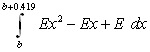Cowculus 2
Grafton Mystery Park Letterbox

 Terrain: Easy - a walk through town and park Difficulty: Need to know Calculus... Created by: The Gals of KML's 06-07 AP Calculus class Placed by: The Dragon (Mathman) Location: Grafton, Ozaukee County Materials needed: Rubber stamp, stamp pad/inking pens, calculator, compass, cluesThis box is the first in a series of letterboxes with Calculus clues.  Thanks to Z-fan for the idea...

Students and parents of students should read my introduction to letterboxing before seeking the boxes.

Although these clues do not use matrices, the graphics calculator simulator for download on this page performs much of the calculus in these clues.  Here is a guidebook on how to use the calculator, in which the calculus commands are explained (see chapter 2).

Start at Walters Dr. and Maple St. in Grafton

1.      A particle is moving along the x-axis with position function s(t) = t2 - 8t + 6 for t > 0 sec.  Find which direction the particle is moving first, and which direction it is moving second.  Facing Walters Dr.(north) with Maple St. going to your left and right, go the direction that the particle is going first.  At the next corner go the direction the particle is moving second.

After walking a few blocks, you will see the park on your left.  Go to the park and find the park sign.  The park closes at a certain time every night.  Remember this number, then go to the big rock near this sign and contemplate the meaning of life while solving this problem:

Let the hour the park closes be t.  Find the value ofThis will give you the bearing to the first stop on your journey to the letterbox.  Walk on this bearing until you reach a place requiring great balance.

3.      Count the number of triangular platforms here: A=__________
(Triangles are everywhere!)

Walk over to the NW corner of the nearby court for competitive games.  Count the number of rings used in the competitive game available here. B=__________

Put this into the equation y = Bx2 - (2B)x + (B-2).
Graph this equation and the line y = A.
Find the area between the curves from (-1, 3).    C=__________

Then find the value of 9C - 12 = D =_____________
Walk on this heading until you reach something the requires a minimum of B people.

4.Walk on this heading until you reach the northwest corner of a maintained area.

5.      Add your first three bearings together and multiply the result by 3.  Then subtract 218.  Let the first two digits be p = _____ (the lower bound) and the last two digits be q = _____ (the upper bound) for the following problem:

Find the area under the graph of y = 3x2 over the interval [p,q].
Walk on this heading until you reach the end of a blue integral.

This next one is a simple, straightforward problem.
Evaluate:= G = _________
Walk on the reverse heading (azimuth) for 16 steps until you reach the middle of something special.
(Hmm, the "reverse" to get to this thing....déjà vu from a Mathman box.

You found E earlier.  Let b be the number of basketball courts in the park.

Evaluate:= ________  (Round to nearest whole number.)
Add 50 to this result.  This will give you the final bearing on which to walk 35 steps to the location of the box.  Dig in an obvious place.  Good luck!

Before you set out read the waiver of responsibility and disclaimer.

LbNA Home